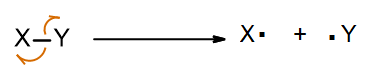# 18.3: Common elementary steps in radical reaction mechanisms

$$\newcommand{\vecs}{\overset { \rightharpoonup} {\mathbf{#1}} }$$ $$\newcommand{\vecd}{\overset{-\!-\!\rightharpoonup}{\vphantom{a}\smash {#1}}}$$$$\newcommand{\id}{\mathrm{id}}$$ $$\newcommand{\Span}{\mathrm{span}}$$ $$\newcommand{\kernel}{\mathrm{null}\,}$$ $$\newcommand{\range}{\mathrm{range}\,}$$ $$\newcommand{\RealPart}{\mathrm{Re}}$$ $$\newcommand{\ImaginaryPart}{\mathrm{Im}}$$ $$\newcommand{\Argument}{\mathrm{Arg}}$$ $$\newcommand{\norm}{\| #1 \|}$$ $$\newcommand{\inner}{\langle #1, #2 \rangle}$$ $$\newcommand{\Span}{\mathrm{span}}$$ $$\newcommand{\id}{\mathrm{id}}$$ $$\newcommand{\Span}{\mathrm{span}}$$ $$\newcommand{\kernel}{\mathrm{null}\,}$$ $$\newcommand{\range}{\mathrm{range}\,}$$ $$\newcommand{\RealPart}{\mathrm{Re}}$$ $$\newcommand{\ImaginaryPart}{\mathrm{Im}}$$ $$\newcommand{\Argument}{\mathrm{Arg}}$$ $$\newcommand{\norm}{\| #1 \|}$$ $$\newcommand{\inner}{\langle #1, #2 \rangle}$$ $$\newcommand{\Span}{\mathrm{span}}$$

Because radical reactions involve structures not seen on “standard” mechanisms (see section 7.5.), the elementary steps involved are different from standard elementary steps (see section 7.6.).  There are four common elementary steps:

### (a) Homolysis

As outlined in the previous section, homolysis involves the splitting of a bond down the middle to produce two radicals.  This is usually how radicals are generated; this means nearly all radical reactions begin with this.This is simply the reverse of homolysis – two unpaired electrons come together and make a bond.  Most radical mechanisms have this as a terminating step – the step that ends a chain reaction.### (c) Bimolecular homolytic substitution (SH2)

This is the radical equivalent of the SN2 step in “normal” mechanisms.  This step is very common in the propagation steps of a radical mechanism – the main part of the mechanism – and it’s usually where the useful bond-making step occurs.